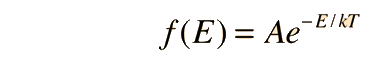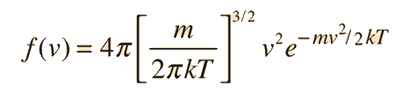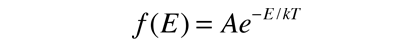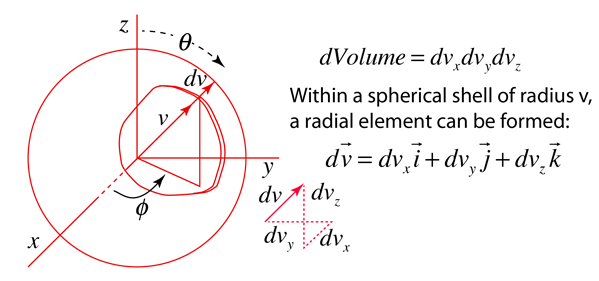# Maxwell Speed Distribution Directly from Boltzmann Distribution

Fundamental to our understanding of classical molecular phenomena is the Boltzmann distribution, which tells us that the probability that any one molecule will be found with energy E decreases exponentially with energy; i.e., any one molecule is highly unlikely to grab much more than its average share of the total energy available to all the molecules. Mathematically, the Boltzmann distribution can be written in the formThis distribution can be made plausible by a numerical example, particularly when put in graphical form, but the rigorous mathematical development by Boltzmann still stands as a major achievement in the mathematics of physics. We will take it as a postulate here and show that the Maxwell speed distribution follows from it.

If this distribution is applied to one direction of velocity for a molecule in an ideal gas, it becomesShow

Converting this relationship to one which expresses the probability in terms of speed in three dimensions gives the Maxwell speed distribution:The steps involved in this conversion are

 Conversion to three-dimensional probability Summing over all spatial directions
Index

Kinetic theory concepts

 HyperPhysics***** Heat and Thermodynamics R Nave
Go Back

# Velocity Distribution in One Dimension

If the energy in the Boltzmann distributionis just one-dimensional kinetic energy, then the expression becomesBut this must be normalized so that the probability of finding it at some value of velocity is one. This is accomplished by integrating the probability from minus to plus infinity and setting it equal to one. Making use of the definite integral formwith the substitutionallows us to normalize the function:This normalizes the distribution function toDiscussion of Maxwell speed distribution development
Index

Kinetic theory concepts

 HyperPhysics***** Heat and Thermodynamics R Nave
Go Back

# Kinetic Energy Distribution in Three Dimensions

We have shown that the one-dimensional energy distribution isbut would like to have a distribution for three dimensions. A basic probability idea is that for three independent events you take the product of the individual probabilities. The three-dimensional probability distribution then takes the form:It must be noted here that while this has the form of the Boltzmann distribution for kinetic energy, it does not take into account the fact that there are more ways to achieve a higher velocity. In making the step from this expression to the Maxwell speed distribution, this distribution function must be multiplied by the factor 4πv2 to account for the density of velocity states available to particles.

 Discussion of Maxwell speed distribution development
Index

Kinetic theory concepts

 HyperPhysics***** Heat and Thermodynamics R Nave
Go Back

# Speed distribution as a sum over all directions

To put the three-dimensional energy distribution into the form of the Maxwell speed distribution, we need to sum over all directions. One way to visualize that sum is as the development of a spherical shell volume element in "velocity space".The sum over the angular coordinates is just going to give the area of the sphere, and the radial element dv gives the thickness of the spherical shell. That takes the angular coordinates out of the distribution function and gives a one-parameter distribution function in terms of the "radial" speed element dv.An alternate, more directly mathematical approach is to make the conversion of the cartesian volume element to spherical polar coordinates.Discussion of Maxwell speed distribution development
Index

Kinetic theory concepts

 HyperPhysics***** Heat and Thermodynamics R Nave
Go Back